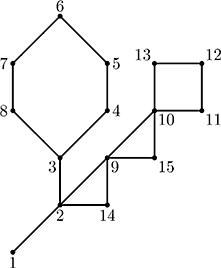Online JudgeProblem SetAuthorsOnline ContestsUser
Web Board
F.A.Qs
Statistical Charts
Problems
Submit Problem
Online Status
Prob.ID:
Register
Authors ranklist
Current Contest
Past Contests
Scheduled Contests
Award Contest
Register
Language:
 Time Limit: 5000MS Memory Limit: 65536K Total Submissions: 1289 Accepted: 416 Special Judge

Description

Cactus is a connected undirected graph in which every edge lies on at most one simple cycle. Intuitively cactus is a generalization of a tree where some cycles are allowed. Your task is to find a diameter of the given cactus. Diameter is the maximal length of the shortest path between pairs of vertices.For example, on the picture above the shortest path between vertices 6 and 12 goes through 8 edges and it is the maximal shortest path in this graph, thus its diameter is 8.

Input

The first line of the input file contains two integer numbers n and m (1 ≤ n50 000, 0 ≤ m10 000). Here n is the number of vertices in the graph. Vertices are numbered from 1 to n. Edges of the graph are represented by a set of edge-distinct paths, where m is the number of such paths.

Each of the following m lines contains a path in the graph. A path starts with an integer number ki (2 ≤ ki ≤ 1000) followed by ki integers from 1 to n. These ki integers represent vertices of a path. Adjacent vertices in a path are distinct. Path can go to the same vertex multiple times, but every edge is traversed exactly once in the whole input file. There are no multiedges in the graph (there is at most one edge between any two vertices).

The graph in the input file is a cactus.

Output

Write to the output file a single integer number — the diameter of the given cactus.

Sample Input

```15 3
9 1 2 3 4 5 6 7 8 3
7 2 9 10 11 12 13 10
5 2 14 9 15 10```

Sample Output

`8`

Source

[Submit]   [Go Back]   [Status]   [Discuss]Home PageGo BackTo top